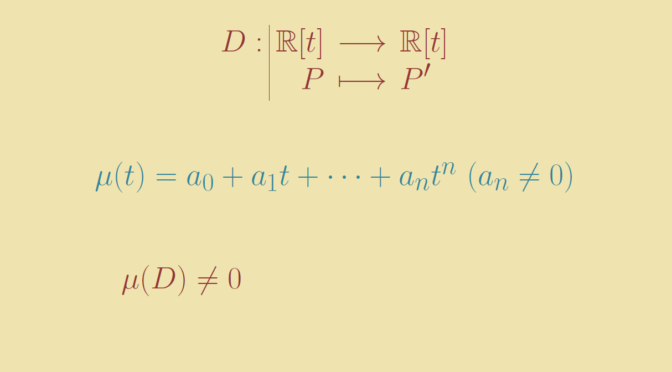A linear map without any minimal polynomial

Given an endomorphism $$T$$ on a finite-dimensional vector space $$V$$ over a field $$\mathbb F$$, the minimal polynomial $$\mu_T$$ of $$T$$ is well defined as the generator (unique up to units in $$\mathbb F$$) of the ideal:$I_T= \{p \in \mathbb F[t]\ ; \ p(T)=0\}.$

For infinite-dimensional vector spaces, the minimal polynomial might not be defined. Let’s provide an example.

We take the real polynomials $$V = \mathbb R [t]$$ as a real vector space and consider the derivative map $$D : P \mapsto P^\prime$$. Let’s prove that $$D$$ doesn’t have any minimal polynomial. By contradiction, suppose that $\mu_D(t) = a_0 + a_1 t + \dots + a_n t^n \text{ with } a_n \neq 0$ is the minimal polynomial of $$D$$, which means that for all $$P \in \mathbb R[t]$$ we have $a_0 + a_1 P^\prime + \dots + a_n P^{(n)} = 0.$ Taking for $$P$$ the polynomial $$t^n$$ we get $a_0 t^n + n a_1 t^{n-1} + \dots + n! a_n = 0,$ which doesn’t make sense as $$n! a_n \neq 0$$, hence $$a_0 t^n + n a_1 t^{n-1} + \dots + n! a_n$$ cannot be the zero polynomial.

We conclude that $$D$$ doesn’t have any minimal polynomial.